# Parallel Viscous Force Densities

It is helpful to define the average fraction of passing particles on a magnetic flux-surface: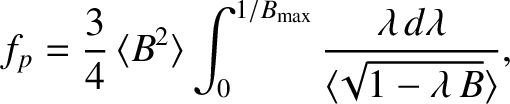(2.200)

where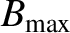is the maximum value of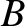on the surface . For a flux-surface with a circular poloidal cross-section ,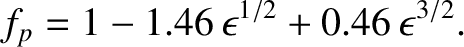(2.201)

Thus, the average fraction of trapped particles on the flux-surface is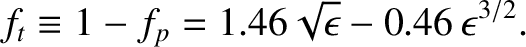(2.202)

Note that the previous expression differs slightly from the less exact expression (2.87).

In the so-called banana collisionality regime,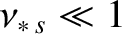[see Equation (2.95)], the flux-surface averaged parallel viscous force densities take the form [22,34,43]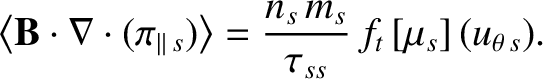(2.203)

The normalized viscosity coefficients for the ions are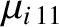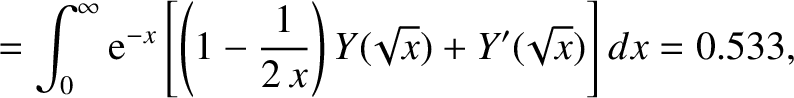(2.204)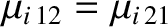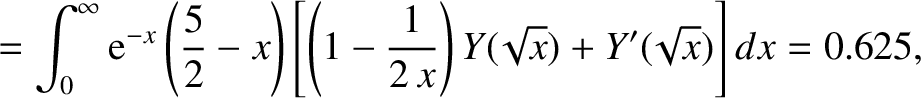(2.205)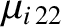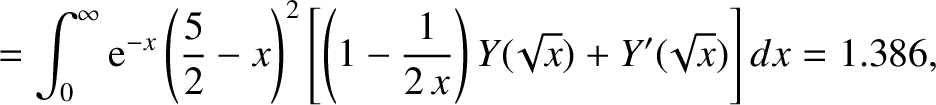(2.206)

where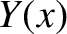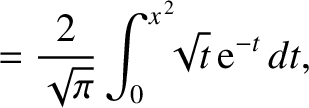(2.207)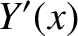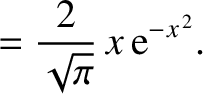(2.208)

The normalized viscosity coefficients for the electrons are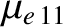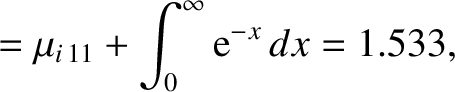(2.209)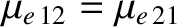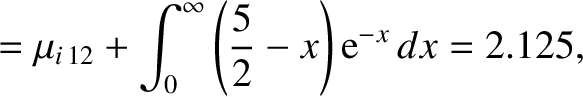(2.210)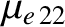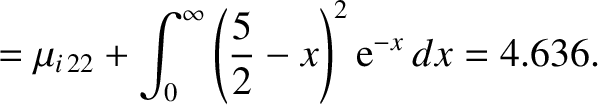(2.211)

In the banana collisionality regime, the parallel viscous force arises from collisional drag between passing and trapped particles [22,43]. The origin of this drag is the fact that, while passing particles can drift along magnetic field-lines in one direction, trapped particles are forced to periodically reverse direction. Not surprisingly, the viscous force density is proportional to the fraction of trapped particles,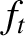. [See Equation (2.203).]